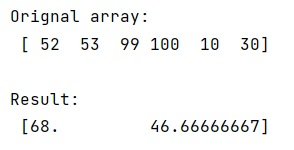# Averaging over every n elements of a NumPy array

Learn, how to average over every n elements of a NumPy array in Python?
Submitted by Pranit Sharma, on January 12, 2023

NumPy is an abbreviated form of Numerical Python. It is used for different types of scientific operations in python. Numpy is a vast library in python which is used for almost every kind of scientific or mathematical operation. It is itself an array which is a collection of various methods and functions for processing the arrays.

## NumPy Array - Averaging over every n elements

Suppose that we are given a NumPy array and we need to create a new array which is the average over every consecutive triplet of elements so that the new array will be one-third of the size as the original.

If the length of the array is divisible by 3, using the numpy.mean() method would be a better option where we will reshape the array by taking 3 columns of a row at a time.

Let us understand with the help of an example,

## Python code for averaging over every n elements of a NumPy array

```# Import numpy
import numpy as np

# Creating numpy array
arr = np.array([52,53,99,100,10,30])

# Display original array
print("Orignal array:\n",arr,"\n")

# Calculating mean
res = np.mean(arr.reshape(-1, 3), axis=1)

# Display result
print("Result:\n",res)
```

Output: